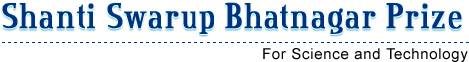• |• Home
• Brief Profile of the Awardee

Brief Profile of the AwardeeDrNitin Saxena

• 2018
• Mathematical Sciences
• 03/05/1981
• Algebraic Complexity Theory
Award Citation:

Dr Saxena has made outstanding work on the Polynomial Identity Testing approach in the field of complexity theory.

 NA
 NA
 NA
 NA
(a) Significant contributions to science and/ or technology development by the nominee based on the work done in India during most part of last 5 years:
During 2013-18 Nitin's focus has been to develop zero testing (or PIT) algorithms for algebraic circuits. This is a computational algebra problem with numerous practical applications and a beautiful theory (eg. primality testing & graph matching are solved using PIT); but more importantly, it strongly relates to showing that there are explicit/ natural polynomials that require exponentially large circuits. The latter is also called the algebraic version of the P≠NP question; P≠?NP is one of the highly-recognized ``Clay Mathematics Institute's Millennium Problems'' and lies in the intersection of Mathematics & Computing. With this motivation, Nitin investigates the algebraic model of computation. Nitin invented the concept of rank-concentration (STOC 2013 pg.321-330) and pioneered those of algebraic-dependence (Information & Computation 2013, 222 pg.2-19), (SIAM J.Comput. 9 2016, 45(4) pg.1533–1562), incidence-geometry (J.ACM 2013 art.33) and duality (Comput.Complexity 2013, 22(1) pg.39-69). These works became well-established in the community and the tools were further developed by various researchers. Nitin himself was instrumental in improving rank concentration for read-once algebraic branching programs (SIAM J.Comput. 2015, 44(3) pg.669-697); developing the best known hitting-sets for the sum-of-ROABPs (Comput.Complexity 2017, 26(4) pg.835-880), constant-width ROABP (Theory of Computing 2017, 13(999) pg.1-21) and log-variate polynomials (Preprint 2018). Recently, he has discovered a phenomenon called `bootstrapping of variables' that reduces the PIT question to that of very simple polynomials (50th STOC 2018). This opens up a new regime where nontrivial PIT algorithms are yet to be found; but once we find them, it will imply general PIT & lower bound results. Nitin introduced algebraic dependence (AD) as a tool useful in PIT algorithms; but, this has become a concept of independent interest hence. He discovered the first nontrivial criterion for AD over finite fields using p-adic cohomology methods (Trans.Amer.Math.Soc. 2014, 366(7) pg.3425- 3450). He devised another criterion using I-adic methods (MFCS 2016 pg.74:1-74:15 & Comput.Complexity 2018) that also yields new PIT algorithms and exponential circuit lower bounds over finite fields. Recently, using algebraic-geometry methods, Nitin showed that AD is a problem that is unlikely to be NP-hard (Preprint 2018). Surprisingly, the methods extend to solve an open problem (posed by Mulmuley in Journal of the American Mathematical Society, 30(1), 2017) about Noether Normalization maps & the parameters of the invariant ring of an explicit variety. In fact, a more general problem of polynomial satisfiability in infinitesimal approximative complexity gets solved unexpectedly in polynomial space, while the earlier best was an exponential space algorithm using Elimination Theory. Nitin developed novel methods for factoring polynomials and integers. He showed that association schemes can be used to factor polynomials under the generalized Riemann hypothesis (LMS J.Comput.Math. 2014, 17(1) 123-140) and Stickelberger property can be used to potentially eliminate the need of GRH in factoring (ISSAC 2017). Recently, using I-adic methods, he showed that a certain family of exponential-degree small circuits have small low-degree factors (50th STOC 2018). This is a natural family and size-bounds for its low-degree factors was an open problem. The work also proves the first nontrivial factor-size bounds for formulas and algebraic branching programs. He has shown that integers can be factored if one can guess algebraic dependencies among its factors (LiPiCS, MFCS 2016 pg.6:1-6:14)
(b) Impact of the contributions in the field concerned:
Algebra or complexity theory each has a long and fruitful history; but, algebraic complexity theory has seen an `intense revival' in this decade. Many of the famous results in this area have been proved by Nitin in his coauthored papers, where numerous new algebraic tools have been invented. He has been a global leader in championing a wide variety of problems-- blackbox identity testing (or VP≠VNP 10 question), root finding, algebraic dependence, algebra isomorphism, and primality testing. The results have been used, and formally cited in excess of 2400 times (scholar.google.com), by the global research community. These results have an immediate impact of enriching the body of algebra, and will have a longer term impact on the design of algebraic algorithms and algebraic circuit lower bound proofs. Computational algebra/ algebraic-geometry/ number-theory are already used in many practical applications. The rapidly growing theory of algebraic complexity provides stronger mathematical foundation to the practice of computing (and vice versa!). This is where I believe Nitin's contributions impact in a big way.
 NA
 NA
 NA
 NA
 NA
 NA
 NA
 NA
 NA
 NA
 NA
 NA
 NA
 NA

Contact Details

• Department of Computer Science and Engineering
Indian Institute of Technology Kanpur

Kanpur - 208016